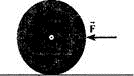Problem

# A large barrel lies on its side. In order to roll it across the floor, you apply a horizon...

A large barrel lies on its side. In order to roll it across the floor, you apply a horizontal force, as shown in the figure. If the applied force points toward the axis of rotation, which runs down the center of the barrel through the center of mass, it produces zero torque about that axis. How then can this applied force make the barrel start to roll?#### Step-by-Step Solution

Solution 1

Torque $$\vec{\tau}=\vec{r} \times \vec{F}$$

When the force acts along the center of the axis of the rotation and through the center of mass then torque acting on the barrel is zero .because the perpendicular distance between axis of rotation and force is zero.

If the force is applied at the edge of the barrel then the perpendicular distance between axis of rotation and force is maximum, and the angle between them is $$90^{\circ}$$. hence the torque acting on it maximum and it can roll.